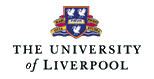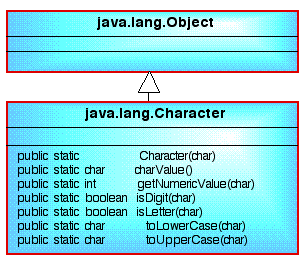# NOTE: This set of www pages is not the set of www pages for the curent version of COMP101. The pages are from a previous version that, at the request of students, I have kept on line.

## CONTENTS

 1. Introduction 2. ASCII Code 3. Alternative codes 4. The Character class 5. Character input 6. Example problem - lower to upper case conversion ` ` 6.1. Requirements 6.2. Analysis 6.3. Design 6.4. Implementation 6.5. Testing 7. The System.out.flush method

Example combines the two statement input data declaration used up until now, into a single statement declaration. Example also introduces the concepts of Boundary Value Analysis (BVA) and limit testing.

## 1. INTRODUCTION

The Java type character is used for handling single characters such as letters, digits and special symbols (e.g. question mark, full stop, colon etc.), or non-printable control character (e.g. tab, newline etc.). In Java (like many other programming languages) characters are written by enclosing them in single quotes. Examples:

```'a'     'A'     '2'     '+'     '''
```

## 2. ASCII CODE

In the early days of computing characters were usually stored, in a computer, using a group of 8 bits, i.e. a byte. Originally, only seven of these bits were used. The eighth most significant bit, referred to as the parity bit, was used for error checking. Using only seven bits there are 128 different character codes available (2^7). There is a generally accepted standard, called the ASCII standard, which determines which characters can be encoded using the seven available bits, and which character code represents which character. ASCII (pronounced "ass-key") is an acronym for American Standard Code for Information Interchange.

## 3. ALTERNATIVE CODES

The ASCII standard was developed on the assumption that all computer usage would be in English. The English alphabet has 26 letters derived from the Latin alphabet. This set of letters is sufficient for only a small group of languages, e.g. English, Swahili and Hawaiian! All other living languages use either the Latin alphabet plus other characters, or other non-Latin alphabets, or syllabaries. Use of the ASCII standard therefore presents a problem in many countries.

### 3.1 LATIN-1 CODE

The obvious solution to addressing the above problem is to drop the use of the parity bit so that 256 character codes are available. There are a number of "8 bit" character standards available. Some languages (for example Ada) use what is commonly referred to as the LATIN-1 standard (ISO-8859). In this standard the first 128 codes (0 to 127) adhere to the ASCII standard, while the remaining codes provide for additional characters.

### 3.2 Unicode Worldwide Character Standard

The Unicode Worldwide Character Standard is a character coding system whereby characters are stored in two bytes of memory (i.e. 16 bits as opposed to 8 bits). "At time of writing" the Unicode standard contained 34,168 distinct coded characters. Java use the Unicode Standard.

Provided that we have an editor that supports the Unicode character set we can include any of the Unicode characters in our Java programs.

## 4. THE Character CLASS

 The character class contains many useful methods for manipulating and testing characters. A Fragment of this class is presented in Figure 1. This fragment includes the following: Character Constructor to create an instance of the class Character so that it represents the primitive value given as its argument. charValue Returns the value of an instance of the class Character. getNumericValue returns the Unicode numeric value of the character as a non-negative integer. isDigit determines if the specified character is a digit (a number). isLetter determines if the specified character is a letter. toLowerCase maps the given character to its lowercase equivalent; if the character has no lowercase equivalent, the character itself is returned. toUpperCase converts the character argument to uppercase. ` `Figure 1: Class diagram for Character class Note: the above five functions are all class methods so are invoked by linking the desired method to the class name Character, e.g.: ```Character.isLetter(n); ``` where n is a data item of type char. Note also that the Character class contains many methods of the form is... for carrying out various test on instances of the type Character.

## 5. CHARACTER INPUT

 Input, using the next method in the Scanner class is always in the form of a string. If, for example, we want integers or doubles we use the nextInt or nextDouble methods respectively. However there is no "nextChar" method. There are mechanisms for getting a single "char" from the input stream but at present we do not have sufficient knowledge to do this. However, what we can do is input a charcter as an ASCII integer and convert it to a "char" using a cast. Thus: ```char inputInt = input.next(); char inputChar = (char) inputInt; ``` where input (in input.next()) is an instance of the Scanner class. Of course we can run the two statments together as follows: ```char inputChar = (char) input.next(); ``` The code example presented in Table 1 indicates how two characters may be input.
 ```// CHARACTER INPUT APPLICATION // Frans Coenen // Thursday 3 August 2000 // Revised: Wednesday 30 June 2005 to be compatible with Java 1.5 // The University of Liverpool, UK import java.util.*; class CharacterInputApp { // ------------------- FIELDS ------------------------ // Create Scanner class instance private static Scanner input = new Scanner(System.in); // ------------------ METHODS ------------------------ public static void main(String[] args) { // Invite input System.out.println("Input two characters seperated by a " + "carriage return:"); // Read in input as a string. char inputChar1 = (char) input.nextInt(); char inputChar2 = (char) input.nextInt(); // Output the result System.out.println("input 1 = " + inputChar1 + " input 2 = " + inputChar2); } } ```

Table 1: Character input code example

## 7. THE System.out.flush METHOD

 When using System.out.print() to output data the data is first passed to a temporary storage area called a buffer from where it is output to (say) the screen. This arrangement is known as output buffering and is designed to save processing time, however it may cause code to appear to be behaving in a strange manner. This is because output is not always passed from the buffer to the screen immediately; the Java interpreter might process some further lines of code before doing this. To force the buffer to be flushed we can use the method: ```System.out.flush(); ``` ` ` contained in the PrintStream and PrintWriter classes. For exmple we might write: ```System.out.print("Answer = "); System.out.flush(); System.out.print(100/5); ``` This will cause the string "Answer = " to be output before the calculation is undertaken. The buffer is always flushed whenever a "new line" character is encountered. Therefore when using System.out.println() the above is not a problem.

Created and maintained by Frans Coenen. Last updated 10 February 2015